# HSPT Math : How to find surface area

## Example Questions

← Previous 1 3

### Example Question #1 : How To Find Surface Area

A right circular cylinder has a height of 41 in. and a lateral area (excluding top and bottom) 512.5π in2. What is the area of its bases?

156.25 in2

78.125π in2

39.0625π in2

312.5 in2

78.125π in2

Explanation:

The lateral area (not including its bases) is equal to the circumference of the base times the height of the cylinder. Think of it like a label that is wrapped around a soup can. Therefore, we can write this area as:

A = h * π * d or A = h * π * 2r = 2πrh

Now, substituting in our values, we get:

512.5π = 2 * 41*rπ; 512.5π = 82rπ

Solve for r by dividing both sides by 82π:

6.25 = r

From here, we can calculate the area of a base:

A = 6.252π = 39.0625π

NOTE: The question asks for the area of the bases. Therefore, the answer is 2 * 39.0625π or 78.125π in2.

### Example Question #1 : How To Find Surface Area

The number of square units in the surface area of a right circular cylinder is equal to the number of cubic units in its volume. If r and h represent the length in units of the cylinder's radius and height, respectively, which of the following is equivalent to r in terms of h?

r = h/(2h – 2)

r = h2 + 2h

r = 2h/(h – 2)

r = 2h2 + 2

r = h2/(h + 2)

r = 2h/(h – 2)

Explanation:

We need to find expressions for the surface area and the volume of a cylinder. The surface area of the cylinder consists of the sum of the surface areas of the two bases plus the lateral surface area.

surface area of cylinder = surface area of bases + lateral surface area

The bases of the cylinder will be two circles with radius r. Thus, the area of each will be πr2, and their combined surface area will be 2πr2.

The lateral surface area of the cylinder is equal to the circumference of the circular base multiplied by the height. The circumferece of a circle is 2πr, and the height is h, so the lateral area is 2πrh.

surface area of cylinder = 2πr2 + 2πrh

Next, we need to find an expression for the volume. The volume of a cylinder is equal to the product of the height and the area of one of the bases. The area of the base is πr2, and the height is h, so the volume of the cylinder is πr2h.

volume = πr2h

Then, we must set the volume and surface area expressions equal to one another and solve for r in terms of h.

2πr2 + 2πrhπr2h

First, let's factor out 2πr from the left side.

2πr(h) = πr2h

We can divide both sides by π.

2r(h) = r2h

We can also divide both sides by r, because the radius cannot equal zero.

2(h) = rh

Let's now distribute the 2 on the left side.

2r + 2h = rh

Subtract 2r from both sides to get all the r's on one side.

2h = rh – 2r

rh – 2r = 2h

Factor out an r from the left side.

r(h – 2) = 2h

Divide both sides by h – 2

r = 2h/(h – 2)

The answer is r = 2h/(h – 2).

### Example Question #2 : How To Find The Surface Area Of A Cylinder

What is the surface area of a cylinder with a radius of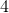and a height of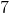?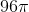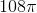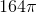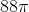Explanation:

When you're calculating the surface area of a cylinder, note that the cylinder will have two circles, one for the top and one for the bottom, and one rectangle that wraps around the "side" of the cylinder (it's helpful to picture peeling the label off a can of soup - it's curved when it's on the can, but really it's a rectangle that has been wrapped around).  You know the area of the circle formula; for the rectangle, note that the height is given to you but the width of the rectangle is one you have to intuit: it's the circumference of the circle, because the entire distance around the circle from one point around and back again is the horizontal distance that the area must cover.

Therefore the surface area of a cylinder =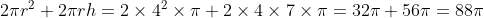### Example Question #1 : How To Find Surface Area

What is the surface area of a cube with a volume of 1728 in3?

1728 in2

72 in2

864 in2

432 in2

144 in2

864 in2

Explanation:

This problem is relatively simple. We know that the volume of a cube is equal to s3, where s is the length of a given side of the cube. Therefore, to find our dimensions, we merely have to solve s3 = 1728. Taking the cubed root, we get s = 12.

Since the sides of a cube are all the same, the surface area of the cube is equal to 6 times the area of one face. For our dimensions, one face has an area of 12 * 12 or 144 in2. Therefore, the total surface area is 6 * 144 = 864 in2.

### Example Question #1 : How To Find The Surface Area Of A Cube

If the volume of a cube is 216 cubic units, then what is its surface area in square units?

64

36

54

216

108

216

Explanation:

The volume of a cube is given by the formula V =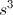, where V is the volume, and s is the length of each side. We can set V to 216 and then solve for s.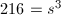In order to find s, we can find the cube root of both sides of the equaton. Finding the cube root of a number is the same as raising that number to the one-third power.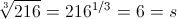This means the length of the side of the cube is 6. We can use this information to find the surface area of the cube, which is equal to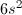. The formula for surface area comes from the fact that each face of the cube has an area of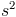, and there are 6 faces in a cube.

surface area =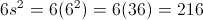The surface area of the square is 216 square units.

### Example Question #710 : Sat Mathematics

You have a cube with sides of 4.5 inches. What is the surface area of the cube?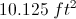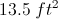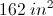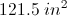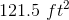Explanation:

The area of one side of the cube is: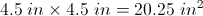A cube has 6 sides, so the total surface area of the cube is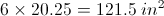### Example Question #711 : Sat Mathematics

A cube has a surface area of 24. If we double the height of the cube, what is the volume of the new rectangular box?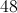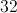Explanation:

We have a cube with a surface area of 24, which means each side has an area of 4. Therefore, the length of each side is 2. If we double the height, the volume becomes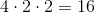.

### Example Question #1 : How To Find The Surface Area Of A Cube

A cube has a surface area of 10m2. If a cube's sides all double in length, what is the new surface area?

20m2

40m2

640m2

80m2

320m2

40m2

Explanation:

The equation for surface area of the original cube is 6s2. If the sides all double in length the new equation is 6(2s)2 or 6 * 4s2. This makes the new surface area 4x that of the old. 4x10 = 40m2

### Example Question #11 : Prisms

A rectangular prism has a volume of 70 m3.  If the length, width, and height of the prism are integers measured in meters, which of the following is NOT a possible measure of the surface area of the prism measured in square meters?

178

174

118

280

214

280

Explanation:

Since the volume is the product of length, width, and height, and each of these three dimensions are integers, it is important to know the factors of the volume.  70 = (2)(5)(7).  This implies that each of these factors (and only these factors with the exception of 1) will show up in the three dimensions exactly once.  This creates precisely the following five possibilities:

2, 5, 7

SA = 2((2)(5)+(2)(7)+(5)(7)) = 118

1, 7, 10

SA = 2((1)(7)+(1)(10)+(7)(10)) = 174

1, 5, 14

SA = 2((1)(5)+(1)(14)+(5)(14)) = 178

1, 2, 35

SA = 2((1)(2)+(1)(35)+(2)(35)) = 214

1, 1, 70

SA = 2((1)(1)+(1)(70)+(1)(70)) = 282

### Example Question #1 : How To Find The Surface Area Of A Prism

The three sides of a rectangular box all have integer unit lengths. If each of the side lengths is greater than one unit, and if the volume of the box is 182 cubic units, what is the surface area of the box in square units?

264

181

262

182

236

262

Explanation:

Let's call the side lengths of the box l, w, and h. We are told that l, w, and h must all be integer lengths greater than one. We are also told that the volume of the box is 182 cubic units.

Since the volume of a rectangular box is the product of its side lengths, this means that lwh must equal 182.

(l)(w)(h) = 182.

In order to determine possible values of l, w, and h, it would help us to figure out the factors of 182. We want to express 182 as a product of three integers each greater than 1.

Let's factor 182. Because 182 is even, it is divisible by 2.

182 = 2(91).

91 is equal to the product of 7 and 13.

Thus, 182 = 2(7)(13).

This means that the lengths of the box must be 2, 7, and 13 units.

In order to find the surface area, we can use the following formula:

surface area = 2lw + 2lh + 2hw.

surface area = 2(2)(7) + 2(2)(13) + 2(7)(13)

= 28 + 52 + 182

= 262 square units.